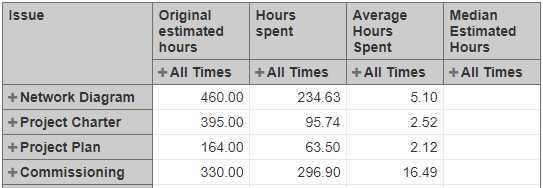# Median of Calculated Members

Hi,

I am creating a report on the number of hours spent on different tasks for different projects. I have grouped the tasks by task type as rows. I was able to find the average of the task types as a column, but I can’t do the same for finding the medians. I tried following this post that has a similar problem that I do, but that solution does not work for me.Here is my current code for Median Estimated Hours:

Median(
Filter(
Descendants([Issue].CurrentMember, [Issue].[Issue]),
[Measures].[Original estimated hours] > 0 AND
[Measures].[Hours spent with sub-tasks] > 0
),
[Measures].[Original estimated hours]
)

It shows the median for each task (which is just itself as it is one value), but I want to get the median time for the whole task type. Does anyone know how to do this?

Hi!

The median calculation is generally correct, only Descendants() function is not working in a case when calculated members of Issue dimension are selected in rows. To retrieve the issue set from a calculated member, use function ChildrenSet() instead.
For more general calculation (to use either of options in rows), you may aggregate both sets and then filter this unified set :

``````{Descendants([Issue].CurrentMember, [Issue].[Issue]),
ChildrenSet([Issue].CurrentMember)}
``````

If Time dimension is used in the report as well, for the sake of optimization when calculating the median of original estimated hours, I would suggest adding additional filter condition by issue creation date property to filter out only issues created in the selected time period (“Original estimated hours” measure is related to issue creation date):

`````` DateInPeriod([Issue].CurrentMember.get('Created at'),
[Time].CurrentHierarchyMember)
``````

So, the final calculation could look the following:

``````Median(
Filter(
{Descendants([Issue].CurrentMember, [Issue].[Issue]),
ChildrenSet([Issue].CurrentMember)},
DateInPeriod([Issue].CurrentMember.get('Created at'),
[Time].CurrentHierarchyMember)
and
[Measures].[Original estimated hours] > 0
and
[Measures].[Hours spent with sub-tasks] > 0
),
[Measures].[Original estimated hours]
)
``````